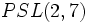# Projective general linear group:PGL(2,7)

(Redirected from PGL(2,7))
View a complete list of particular groups (this is a very huge list!)[SHOW MORE]

## Definition

This group is defined in the following equivalent ways:

1. It is the projective general linear group of degree two over the field of seven elements, i.e., the group$PGL(2,7)$.
2. It is the automorphism group of projective special linear group:PSL(3,2) which in turn is isomorphic to$PSL(2,7)$$PGL(3,2)$,$SL(3,2)$, and$GL(3,2)$.
3. It is the outer linear group$OL(3,2)$.

## GAP implementation

### Group ID

This finite group has order 336 and has ID 208 among the groups of order 336 in GAP's SmallGroup library. For context, there are 228 groups of order 336. It can thus be defined using GAP's SmallGroup function as:

SmallGroup(336,208)

For instance, we can use the following assignment in GAP to create the group and name it$G$:

gap> G := SmallGroup(336,208);

Conversely, to check whether a given group$G$ is in fact the group we want, we can use GAP's IdGroup function:

IdGroup(G) = [336,208]

or just do:

IdGroup(G)

to have GAP output the group ID, that we can then compare to what we want.

### Other definitions

The group can be defined using GAP's ProjectiveGeneralLinearGroup function:

`PGL(2,7)`

It can also be defined using the AutomorphismGroup function on$PSL(3,2)$:

`AutomorphismGroup(PSL(3,2))`